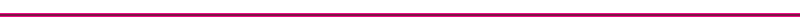Heat Transfer Mechanisms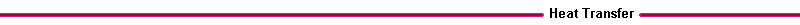The three heat transfer mechanisms are:
Conduction

Conduction heat transfer is energy transport due to molecular motion and interaction. Conduction heat transfer through solids is due to molecular vibration. Fourier determined that Q/A, the heat transfer per unit area (W/m2) is proportional to the temperature gradient dT/dx. The constant of proportionality is called the material thermal conductivity k

Fouriers equation :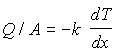The thermal conductivity k depends on the material, for example, the various materials used in engines have the following thermal conductivities (W/m K):

Table I. Thermal Conductives of Common Materials

 Copper 400 Aluminum 240 Cast Iron 80 water 0.61 air 0.026
The thermal conductivity also depends somewhat on the temperature of the material.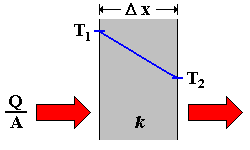Figure 8.  Conduction through Piston Cylinder Wall

For a cast iron 0.012m (½") cylinder block at steady state,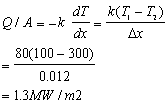The following applet presents a simple conduction example, Conduction Applet.Figure 9.  Boundary Layer

Convection

Convection heat transfer is energy transport due to bulk fluid motion. Convection heat transfer through gases and liquids from a solid boundary results from the fluid motion along the surface.

Newton determined that the heat transfer/area, Q/A, is proportional to the fluid solid temperature difference Ts-Tf. The temperature difference usually occurs across a thin layer of fluid adjacent to the solid surface. This thin fluid layer is called a boundary layer. The constant of proportionality is called the heat transfer coefficient, h.

Newton's Equation: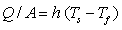The heat transfer coefficient depends on the type of fluid and the fluid velocity. The heat flux, depending on the area of interest, is the local or area averaged. The various types of convective heat transfer are usually categorized into the following areas :

Table II. Convective Heat Transfer Coefficients

Convection type Description Typical value of h (W/m2K)
natural convection fluid motion induced by density differences 10 (gas)

100 (liquid)

forced convection fluid motion induced by pressure differences from a fan or pump 100 (gas)

1000 (liquid)

boiling fluid motion induced by a change of phase from liquid to vapor 20,000
condensation fluid motion induced by a change of phase from vapor to liquid 20,000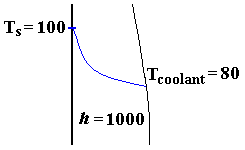Figure 10.  Convection Problem Diagram

For a cylinder block with a forced convection h of 1000, surface temperature of 100C , and a coolant temperature of 80 C, the local heat transfer rate is :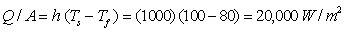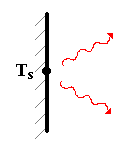Figure11.  Radiation through Piston Cylinder Wall

Radiation heat transfer is energy transport due to emission of electromagnetic waves or photons from a surface or volume. The radiation does not require a heat transfer medium, and can occur in a vacuum. The heat transfer by radiation is proportional to the fourth power of the absolute material temperature. The proportionality constant s is the Stefan-Boltzman constant equal to 5.67 x 10-8 W/m2K4. The radiation heat transfer also depends on the material properties represented by e, the emissivity of the material.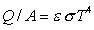For a surface with an emissivity of e = 0.8 and T = 373 K (100C), the radiation heat transfer isFor moderate (less than 100 C) temperature differences, it should be noted that the radiation and natural convection heat transfer are about the same.

The following applet calculates the heat transfered by radiation from a surface at temperature, TsRadiation Applet.